## Modular Function Multiplier

Whenandsatisfy a Modular Equation, a relationship of the form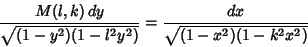(1)

exists, and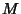is called the multiplier. The multiplier of degreecan be given by(2)

whereis a Theta Function and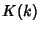is a complete Elliptic Integral of the First Kind.

The first few multipliers in terms ofandare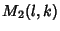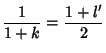(3)(4)

In terms of theand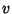defined for Modular Equations,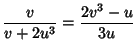(5)(6)(7)